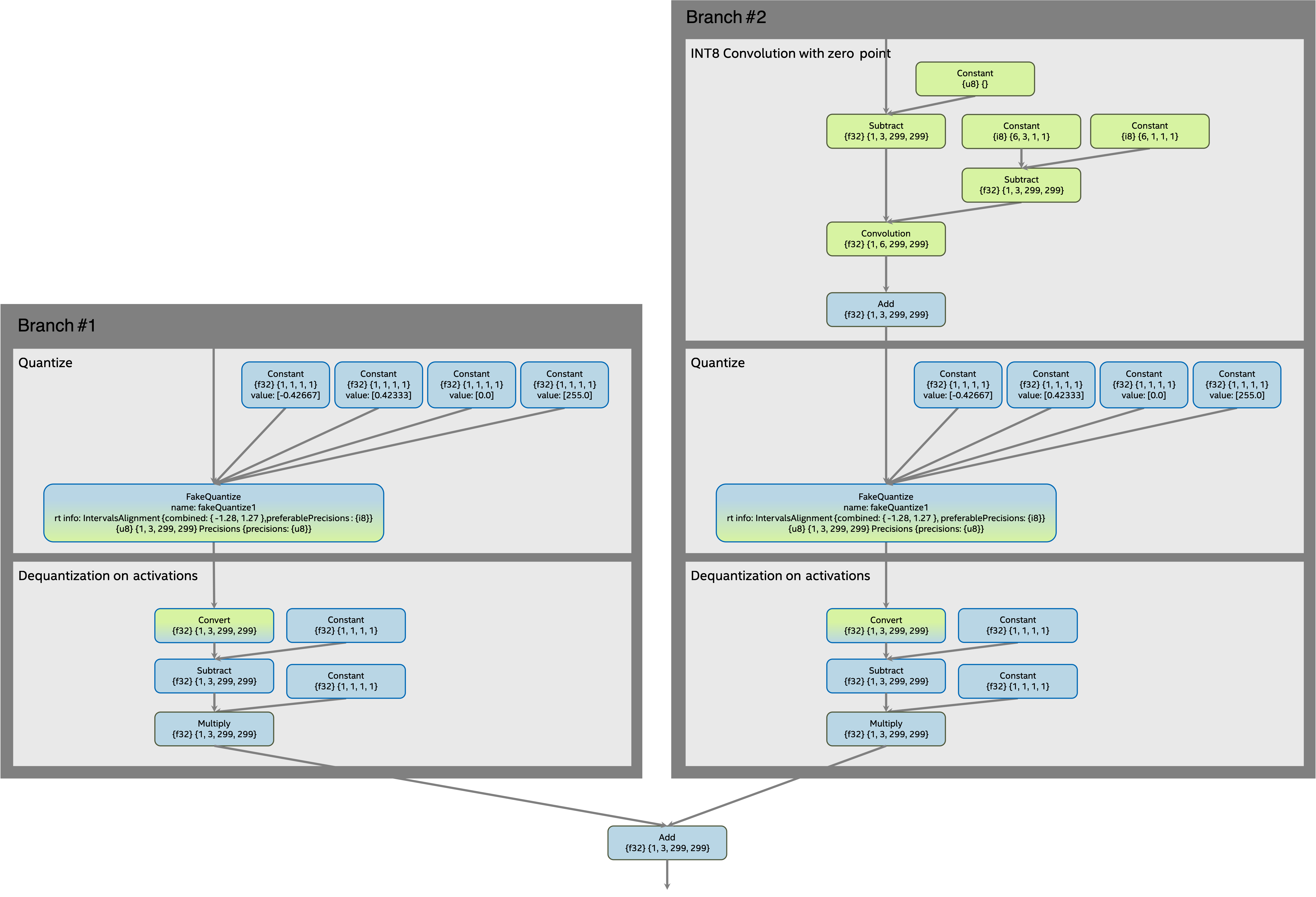ov::pass::low_precision::AddTransformation class represents the Add operation transformation.

The transformation propagates dequantization subtraction from one input branch to another and propagates dequantization multiplication from the same branch through Add operation. In transformation result, one Add operation input branch is in low precision without dequantization operations (empty branch), another input branch is in original precision with updated dequantization operations (full branch).

Criteria for selecting an empty branch in order of priority:

Step 1. If one branch is quantized only, then the quantized branch is an empty branch.

Step 2. If only one branch has FakeQuantize before dequantization operations, then another branch is an empty branch.

Step 3. If some FakeQuantize has more than one consumer and another has only one, then the branch with FakeQuantize with several consumers is an empty branch.

Step 4. Constant branch is in original precision, data branch is an empty branch. In this case, dequantization operations are propagated to a constant branch and will be fused in one constant.

Step 5. If both branches have operations from the following list before FakeQuantize : Convolution, GroupConvolution, and MatMul, or do not have any operations from the list, then the branch with larger shape volume is empty.

Step 6. If the operation before FakeQuantize has several consumers in any branch, then the branch is empty.

If dequantization operations on the full branch have a FakeQuantize operation parent, then they will be fused with FakeQuantize during another low precision transformation. If a FakeQuantize operation has a parent operation from the list: Convolution, GroupConvolution, and MatMul, then during inference the FakeQuantize can be inferred in one plugin kernel with the parent operation.

Depending on the plugin instruction set, low precision inference for the Add operation can be implemented in two logical steps in one plugin kernel:

• Inference step #1: Operations in the full branch, for example, Convolution and FakeQuantize with fused dequantization operations, and Add can be inferred in the original precision.

• Inference step #2: Inference step #1 result can be added with the empty branch tensor in low precision.

This approach allows to infer the Add operation in the optimal way.

## Subgraph before transformation¶

The subgraph with quantized Add operation before transformation:

$y_{ch,i}=(scale1_{ch} \* (x1_{ch,i} - shift1_{ch})) + (scale2_{ch} \* (x2_{ch,i} - shift2_{ch}))$## Subgraph after transformation¶

The subgraph with the Add operation after the transformation:

$y_{ch,i}=scale2_{ch} \* (scale1_{ch}' \* (x1_{ch,i} - shift1_{ch}') + x2_{ch,i})$

where:

$scale1_{ch}' = scale1_{ch} / scale2_{ch}$
$shift1_{ch}' = shift1_{ch} + scale2_{ch} \* shift2_{ch} / scale1_{ch}$